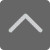• 上海

# 小学口算题大全：二年级100以内加减法口算题（五）

2018-11-02 16:36:41 　来源：网络 文章作者：网络

## — — 学而思小学课程在线预约 — —点击预约→免费的1对1学科诊断及课程规划

40+29+1=

62+15=

54-13=

84+3+7=

68-60=

16+13=

86+4+6=

77-48=

11+17=

46+23+17=

84-1=

18+20=

22+45+15=

61-23=

62+28=

0+55+5=

13+69=

92-3=

77+22+8=

53+18=

20+22=

54+40+10=

89-81=

55+20=

23+25+15=加入QQ群，与更多家长交流经验！！！
 上海小学交流群：639215153 上海初中交流群：611612914 上海高中交流群：959031473 上海幼升小交流群：772707735

### 预约课程更多 >